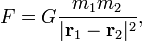# Inverse-square law

In physics, an inverse-square law is any law that states that some quantity is inversely proportional to the square of the distance from some point.

##  Examples

• Isaac Newton's law of universal gravitation states the the gravitational attraction between any two massive objects ("bodies") is directly proportional to the product of their masses and inversely proportional to the square of the distance between them. More precisely, if the center of mass of a body of mass m i is at position r i for i = 1,2, then Newton's gravitational law states that the two bodies attract each other with a force of absolute value,$F = G \frac{m_1 m_2}{|\mathbf{r}_1 - \mathbf{r}_2|^2},$
where the Newtonian constant of gravitation G is 6.674 28 × 10−11 m3kg−1 s−2. Both bodies feel an attraction along the line connecting the centers of mass of the bodies.
• Coulomb's Law states that the attractive (in the case of opposite charges) or repulsive (in the case of like charges) force between any two electrically charged objects is directly proportional to the product of the charges and inversely proportional to the square of the distance between them.Some content on this page may previously have appeared on Citizendium.